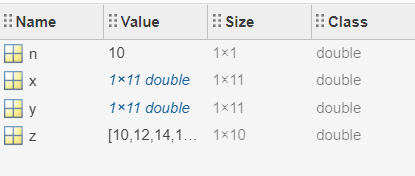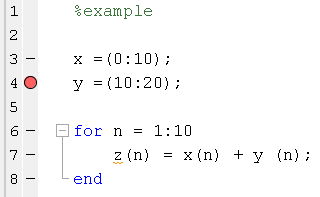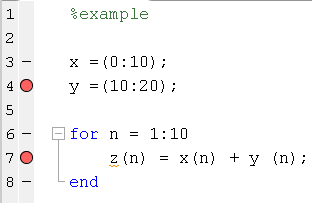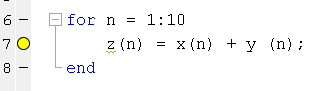Set Breakpoints for Debugging MATLAB Code

• Last Updated : 13 Oct, 2021

MATLAB is one of the most powerful coding languages.MATLAB stands for Matrix Laboratory. It is a high-performance language that is used for technical computing. It was developed by Cleve Molar of the company MathWorks.Inc in the year 1984. It is written in C, C++, Java. It allows matrix manipulations, plotting of functions, implementation of algorithms, and creation of user interfaces. Now let’s talk about an important option in the MATLAB environment, Breakpoints.

Breakpoints are used in the debugging process to evaluate the code and to determine whether a specified line is the source of an error or not.

First of all, MATLAB has three types of breakpoints:

• Standard breakpoints
• Conditional breakpoints
• Error breakpoints

Let’s see into them using an example of a code that generates two arrays then add every two corresponding elements and save them into a new array:

Example:

Matlab

 % MATLAB code for without breakpoints% define x and yx = (0:10);y = (10:20);  for n = 1:10    z(n) = x(n) + y (n);end

Output:Standard breakpoints

They are the common type of breakpoints that simply pauses at specific lines execution, to set them: first of all, you should save your file, also you should check for syntax error as they prevent breakpoints from being saved. Here are some ways to insert standard breakpoints:

Method 1:

Step 1: Click on the dash in the gray area beside the executable code, a red dot should appear, say we clicked on the dash beside line 4:

Output:Step 2: Put the cursor at the desired line and then press F12.

Method 2:

Programmatically, type in the command window:

Syntax:

dbstop in filename at linenumber

Example:

Matlab

 dbstop in example at line 7

Output:Here, you can not add a breakpoint at the start or end of a loop, instead, you can put them inside the loop to pause at every iteration of the loop.

Conditional breakpoints

They are breakpoints that pause at a line only if a specific condition is met. Programmatically, type in the command window:

Syntax:

dbstop in filename at linenumber condition

Suppose, if you want the for loop code execution after specific iterations, here are some ways to do so:

Step 1: Right-click on the gray area beside the desired line and select ” set conditional breakpoint “.

Step 2: Input the specific condition ( for instance: n>2).

Step 3: Then click OK. A yellow dot should appear instead of the dashed line.

Example:

Matlab

 dbstop in example at 7 if n>2

Output:Error breakpoints

Unlike other breakpoints, you do not set error breakpoints at specified lines. Error breakpoints are simply conditions at which the program pauses at the line where the error occurs, to set them:

Step 1: Click the down the arrow at the RUN button then you can choose:

• Pause on errors: to pause on all errors.
• Pause on warnings: to pause on all warnings.
• Pause on NaN or Inf: to pause on NaN (  not-a-number ) or infinite values.

Programmatically, in the command window insert error breakpoints for a pause on all errors.

Example:

Matlab

 % MATLAB code for dbstop% error breakpoint % define x and yx = (0:10);y = (10:20);dbstop if error for n = 1:10    z(n) = x(n) + y (n);end

Notes:

• A gray breakpoint indicates an invalid breakpoint.
• To disable/clear a breakpoint: right-click on it and choose disable/clear breakpoint.
• To clear all breakpoints: right-click on the grey area and select clear all breakpoints in the file.

My Personal Notes arrow_drop_up